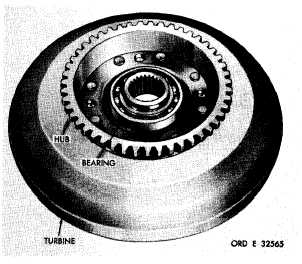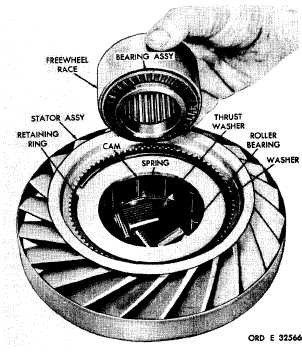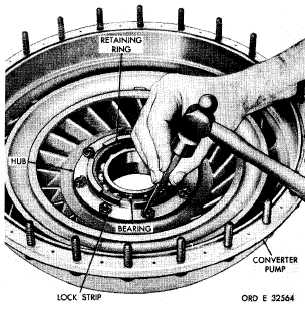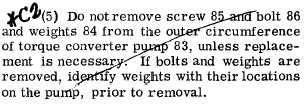S e c t i o n   I V .   T O R Q U E   C O N V E R T E R  R E B U I LD cont.
Custom Search*C2 CHAP   5,   SEC   I V . T O R Q U E     C O N V E R T E R     R E B U I L D P A R    8   3 . P u m p (1)   Straighten   the   tabs   of   lock   strips   on the   converter   pump   hub   (fig.   145). ( 2 )    U s i n g    a    7 / 1 6 - i n c h    w r e n c h ,    r e m o ve e i g h t    s e l f - l o c k i n g    b o l t s    a n d    f o u r    l o c k    s t r i ps retaining   the   torque   converter   pump   hub   in   the converter   pump.   Remove   the   hub. (3)   Remove   two   hook-type   seal   rings   87 (fig.   373,   fold-out   2)   from   pump   nub   88. (4)   Remove   retaining   ring   92   retaining bearing   91   in   converter   pump   hub   88.   Press the   bearing   from   the   hub. c .    T o r q u e    C o n v e r t e r    T u r b i ne (1)   Do   not   remove   the   ball   bearing   from t h e    t u r b i n e    a s s e m b l y ,    u n l e s s    r e p l a c e m e n t    is n e c e s s a r y    ( f i g .    1 4 6 ). Figure 145. Straightening corners of converter pump hub lock strip (2)   If   necessary   to   remove   the   bearing, pry   it   from   the   turbine   hub. .   T o r q u e    C o n v e r t e r    S t a t or (1)   Rotating   the   stator   freewheel   race in   a   clockwise   direction,   remove   it   from   the stator   assembly   (fig.   147). Figure 146. Torque converter turbine assembly Figure 147. Removing stator freewheel race and thrust bearing assembly from stator 1 0 6Integrated Publishing, Inc. - A (SDVOSB) Service Disabled Veteran Owned Small Business